# Gradient Boosted Trees: Model understanding

For an end-to-end walkthrough of training a Gradient Boosting model check out the boosted trees tutorial. In this tutorial you will:

• Learn how to interpret a Boosted Trees model both locally and globally
• Gain intution for how a Boosted Trees model fits a dataset

## How to interpret Boosted Trees models both locally and globally

Local interpretability refers to an understanding of a model’s predictions at the individual example level, while global interpretability refers to an understanding of the model as a whole. Such techniques can help machine learning (ML) practitioners detect bias and bugs during the model development stage.

For local interpretability, you will learn how to create and visualize per-instance contributions. To distinguish this from feature importances, we refer to these values as directional feature contributions (DFCs).

For global interpretability you will retrieve and visualize gain-based feature importances, permutation feature importances and also show aggregated DFCs.

You will be using the titanic dataset, where the (rather morbid) goal is to predict passenger survival, given characteristics such as gender, age, class, etc.

pip install statsmodels

import numpy as np
import pandas as pd
from IPython.display import clear_output

y_train = dftrain.pop('survived')
y_eval = dfeval.pop('survived')

import tensorflow as tf
tf.random.set_seed(123)


For a description of the features, please review the prior tutorial.

## Create feature columns, input_fn, and the train the estimator

### Preprocess the data

Create the feature columns, using the original numeric columns as is and one-hot-encoding categorical variables.

fc = tf.feature_column
CATEGORICAL_COLUMNS = ['sex', 'n_siblings_spouses', 'parch', 'class', 'deck',
'embark_town', 'alone']
NUMERIC_COLUMNS = ['age', 'fare']

def one_hot_cat_column(feature_name, vocab):
return fc.indicator_column(
fc.categorical_column_with_vocabulary_list(feature_name,
vocab))
feature_columns = []
for feature_name in CATEGORICAL_COLUMNS:
# Need to one-hot encode categorical features.
vocabulary = dftrain[feature_name].unique()
feature_columns.append(one_hot_cat_column(feature_name, vocabulary))

for feature_name in NUMERIC_COLUMNS:
feature_columns.append(fc.numeric_column(feature_name,
dtype=tf.float32))


### Build the input pipeline

Create the input functions using the from_tensor_slices method in the tf.data API to read in data directly from Pandas.

# Use entire batch since this is such a small dataset.
NUM_EXAMPLES = len(y_train)

def make_input_fn(X, y, n_epochs=None, shuffle=True):
def input_fn():
dataset = tf.data.Dataset.from_tensor_slices((X.to_dict(orient='list'), y))
if shuffle:
dataset = dataset.shuffle(NUM_EXAMPLES)
# For training, cycle thru dataset as many times as need (n_epochs=None).
dataset = (dataset
.repeat(n_epochs)
.batch(NUM_EXAMPLES))
return dataset
return input_fn

# Training and evaluation input functions.
train_input_fn = make_input_fn(dftrain, y_train)
eval_input_fn = make_input_fn(dfeval, y_eval, shuffle=False, n_epochs=1)


### Train the model

params = {
'n_trees': 50,
'max_depth': 3,
'n_batches_per_layer': 1,
# You must enable center_bias = True to get DFCs. This will force the model to
# make an initial prediction before using any features (e.g. use the mean of
# the training labels for regression or log odds for classification when
# using cross entropy loss).
'center_bias': True
}

est = tf.estimator.BoostedTreesClassifier(feature_columns, **params)
# Train model.
est.train(train_input_fn, max_steps=100)

# Evaluation.
results = est.evaluate(eval_input_fn)
clear_output()
pd.Series(results).to_frame()


For performance reasons, when your data fits in memory, we recommend use the arg train_in_memory=True in the tf.estimator.BoostedTreesClassifier function. However if training time is not of a concern or if you have a very large dataset and want to do distributed training, use the tf.estimator.BoostedTrees API shown above.

When using this method, you should not batch your input data, as the method operates on the entire dataset.

in_memory_params = dict(params)
in_memory_params['n_batches_per_layer'] = 1
# In-memory input_fn does not use batching.
def make_inmemory_train_input_fn(X, y):
y = np.expand_dims(y, axis=1)
def input_fn():
return dict(X), y
return input_fn
train_input_fn = make_inmemory_train_input_fn(dftrain, y_train)

# Train the model.
est = tf.estimator.BoostedTreesClassifier(
feature_columns,
train_in_memory=True,
**in_memory_params)

est.train(train_input_fn)
print(est.evaluate(eval_input_fn))

INFO:tensorflow:Using default config.
WARNING:tensorflow:Using temporary folder as model directory: /tmp/tmp5m737ngz
INFO:tensorflow:Using config: {'_model_dir': '/tmp/tmp5m737ngz', '_tf_random_seed': None, '_save_summary_steps': 100, '_save_checkpoints_steps': None, '_save_checkpoints_secs': 600, '_session_config': allow_soft_placement: true
graph_options {
rewrite_options {
meta_optimizer_iterations: ONE
}
}
, '_keep_checkpoint_max': 5, '_keep_checkpoint_every_n_hours': 10000, '_log_step_count_steps': 100, '_train_distribute': None, '_device_fn': None, '_protocol': None, '_eval_distribute': None, '_experimental_distribute': None, '_experimental_max_worker_delay_secs': None, '_session_creation_timeout_secs': 7200, '_checkpoint_save_graph_def': True, '_service': None, '_cluster_spec': ClusterSpec({}), '_task_type': 'worker', '_task_id': 0, '_global_id_in_cluster': 0, '_master': '', '_evaluation_master': '', '_is_chief': True, '_num_ps_replicas': 0, '_num_worker_replicas': 1}
INFO:tensorflow:Calling model_fn.
INFO:tensorflow:Done calling model_fn.
INFO:tensorflow:Create CheckpointSaverHook.
WARNING:tensorflow:Issue encountered when serializing resources.
Type is unsupported, or the types of the items don't match field type in CollectionDef. Note this is a warning and probably safe to ignore.
'_Resource' object has no attribute 'name'
Exception ignored in: <function CapturableResource.__del__ at 0x7f50f8597cb0>
Traceback (most recent call last):
File "/tmpfs/src/tf_docs_env/lib/python3.7/site-packages/tensorflow/python/training/tracking/tracking.py", line 269, in __del__
with self._destruction_context():
AttributeError: 'TreeEnsemble' object has no attribute '_destruction_context'
INFO:tensorflow:Graph was finalized.
INFO:tensorflow:Running local_init_op.
INFO:tensorflow:Done running local_init_op.
WARNING:tensorflow:Issue encountered when serializing resources.
Type is unsupported, or the types of the items don't match field type in CollectionDef. Note this is a warning and probably safe to ignore.
'_Resource' object has no attribute 'name'
INFO:tensorflow:Calling checkpoint listeners before saving checkpoint 0...
INFO:tensorflow:Saving checkpoints for 0 into /tmp/tmp5m737ngz/model.ckpt.
WARNING:tensorflow:Issue encountered when serializing resources.
Type is unsupported, or the types of the items don't match field type in CollectionDef. Note this is a warning and probably safe to ignore.
'_Resource' object has no attribute 'name'
INFO:tensorflow:Calling checkpoint listeners after saving checkpoint 0...
INFO:tensorflow:loss = 0.6931472, step = 0
WARNING:tensorflow:It seems that global step (tf.train.get_global_step) has not been increased. Current value (could be stable): 0 vs previous value: 0. You could increase the global step by passing tf.train.get_global_step() to Optimizer.apply_gradients or Optimizer.minimize.
INFO:tensorflow:global_step/sec: 133.109
INFO:tensorflow:loss = 0.34396845, step = 99 (0.753 sec)
INFO:tensorflow:Calling checkpoint listeners before saving checkpoint 153...
INFO:tensorflow:Saving checkpoints for 153 into /tmp/tmp5m737ngz/model.ckpt.
WARNING:tensorflow:Issue encountered when serializing resources.
Type is unsupported, or the types of the items don't match field type in CollectionDef. Note this is a warning and probably safe to ignore.
'_Resource' object has no attribute 'name'
INFO:tensorflow:Calling checkpoint listeners after saving checkpoint 153...
INFO:tensorflow:Loss for final step: 0.32042706.
INFO:tensorflow:Calling model_fn.
WARNING:tensorflow:Trapezoidal rule is known to produce incorrect PR-AUCs; please switch to "careful_interpolation" instead.
WARNING:tensorflow:Trapezoidal rule is known to produce incorrect PR-AUCs; please switch to "careful_interpolation" instead.
INFO:tensorflow:Done calling model_fn.
INFO:tensorflow:Starting evaluation at 2021-06-22T01:21:58
INFO:tensorflow:Graph was finalized.
INFO:tensorflow:Restoring parameters from /tmp/tmp5m737ngz/model.ckpt-153
INFO:tensorflow:Running local_init_op.
INFO:tensorflow:Done running local_init_op.
Exception ignored in: <function CapturableResource.__del__ at 0x7f50f8597cb0>
Traceback (most recent call last):
File "/tmpfs/src/tf_docs_env/lib/python3.7/site-packages/tensorflow/python/training/tracking/tracking.py", line 269, in __del__
with self._destruction_context():
AttributeError: 'TreeEnsemble' object has no attribute '_destruction_context'
INFO:tensorflow:Inference Time : 0.45122s
INFO:tensorflow:Finished evaluation at 2021-06-22-01:21:58
INFO:tensorflow:Saving dict for global step 153: accuracy = 0.81439394, accuracy_baseline = 0.625, auc = 0.86923784, auc_precision_recall = 0.85286695, average_loss = 0.41441453, global_step = 153, label/mean = 0.375, loss = 0.41441453, precision = 0.7604167, prediction/mean = 0.38847554, recall = 0.7373737
WARNING:tensorflow:Issue encountered when serializing resources.
Type is unsupported, or the types of the items don't match field type in CollectionDef. Note this is a warning and probably safe to ignore.
'_Resource' object has no attribute 'name'
INFO:tensorflow:Saving 'checkpoint_path' summary for global step 153: /tmp/tmp5m737ngz/model.ckpt-153
{'accuracy': 0.81439394, 'accuracy_baseline': 0.625, 'auc': 0.86923784, 'auc_precision_recall': 0.85286695, 'average_loss': 0.41441453, 'label/mean': 0.375, 'loss': 0.41441453, 'precision': 0.7604167, 'prediction/mean': 0.38847554, 'recall': 0.7373737, 'global_step': 153}


## Model interpretation and plotting

import matplotlib.pyplot as plt
import seaborn as sns
sns_colors = sns.color_palette('colorblind')


## Local interpretability

Next you will output the directional feature contributions (DFCs) to explain individual predictions using the approach outlined in Palczewska et al and by Saabas in Interpreting Random Forests (this method is also available in scikit-learn for Random Forests in the treeinterpreter package). The DFCs are generated with:

pred_dicts = list(est.experimental_predict_with_explanations(pred_input_fn))

(Note: The method is named experimental as we may modify the API before dropping the experimental prefix.)

pred_dicts = list(est.experimental_predict_with_explanations(eval_input_fn))

INFO:tensorflow:Using config: {'_model_dir': '/tmp/tmp5m737ngz', '_tf_random_seed': None, '_save_summary_steps': 100, '_save_checkpoints_steps': None, '_save_checkpoints_secs': 600, '_session_config': allow_soft_placement: true
graph_options {
rewrite_options {
meta_optimizer_iterations: ONE
}
}
, '_keep_checkpoint_max': 5, '_keep_checkpoint_every_n_hours': 10000, '_log_step_count_steps': 100, '_train_distribute': None, '_device_fn': None, '_protocol': None, '_eval_distribute': None, '_experimental_distribute': None, '_experimental_max_worker_delay_secs': None, '_session_creation_timeout_secs': 7200, '_checkpoint_save_graph_def': True, '_service': None, '_cluster_spec': ClusterSpec({}), '_task_type': 'worker', '_task_id': 0, '_global_id_in_cluster': 0, '_master': '', '_evaluation_master': '', '_is_chief': True, '_num_ps_replicas': 0, '_num_worker_replicas': 1}
INFO:tensorflow:Calling model_fn.
INFO:tensorflow:Done calling model_fn.
INFO:tensorflow:Graph was finalized.
INFO:tensorflow:Restoring parameters from /tmp/tmp5m737ngz/model.ckpt-153
INFO:tensorflow:Running local_init_op.
INFO:tensorflow:Done running local_init_op.
Exception ignored in: <function CapturableResource.__del__ at 0x7f50f8597cb0>
Traceback (most recent call last):
File "/tmpfs/src/tf_docs_env/lib/python3.7/site-packages/tensorflow/python/training/tracking/tracking.py", line 269, in __del__
with self._destruction_context():
AttributeError: 'TreeEnsemble' object has no attribute '_destruction_context'

# Create DFC Pandas dataframe.
labels = y_eval.values
probs = pd.Series([pred['probabilities'] for pred in pred_dicts])
df_dfc = pd.DataFrame([pred['dfc'] for pred in pred_dicts])
df_dfc.describe().T


A nice property of DFCs is that the sum of the contributions + the bias is equal to the prediction for a given example.

# Sum of DFCs + bias == probabality.
bias = pred_dicts['bias']
dfc_prob = df_dfc.sum(axis=1) + bias
np.testing.assert_almost_equal(dfc_prob.values,
probs.values)


Plot DFCs for an individual passenger. Let's make the plot nice by color coding based on the contributions' directionality and add the feature values on figure.

# Boilerplate code for plotting :)
def _get_color(value):
"""To make positive DFCs plot green, negative DFCs plot red."""
green, red = sns.color_palette()[2:4]
if value >= 0: return green
return red

"""Display feature's values on left of plot."""
x_coord = ax.get_xlim()
OFFSET = 0.15
for y_coord, (feat_name, feat_val) in enumerate(feature_values.items()):
t = plt.text(x_coord, y_coord - OFFSET, '{}'.format(feat_val), size=12)
t.set_bbox(dict(facecolor='white', alpha=0.5))
from matplotlib.font_manager import FontProperties
font = FontProperties()
font.set_weight('bold')
t = plt.text(x_coord, y_coord + 1 - OFFSET, 'feature\nvalue',
fontproperties=font, size=12)

def plot_example(example):
TOP_N = 8 # View top 8 features.
sorted_ix = example.abs().sort_values()[-TOP_N:].index  # Sort by magnitude.
example = example[sorted_ix]
colors = example.map(_get_color).tolist()
ax = example.to_frame().plot(kind='barh',
color=colors,
legend=None,
alpha=0.75,
figsize=(10,6))
ax.grid(False, axis='y')
ax.set_yticklabels(ax.get_yticklabels(), size=14)

return ax

# Plot results.
ID = 182
example = df_dfc.iloc[ID]  # Choose ith example from evaluation set.
TOP_N = 8  # View top 8 features.
sorted_ix = example.abs().sort_values()[-TOP_N:].index
ax = plot_example(example)
ax.set_title('Feature contributions for example {}\n pred: {:1.2f}; label: {}'.format(ID, probs[ID], labels[ID]))
ax.set_xlabel('Contribution to predicted probability', size=14)
plt.show()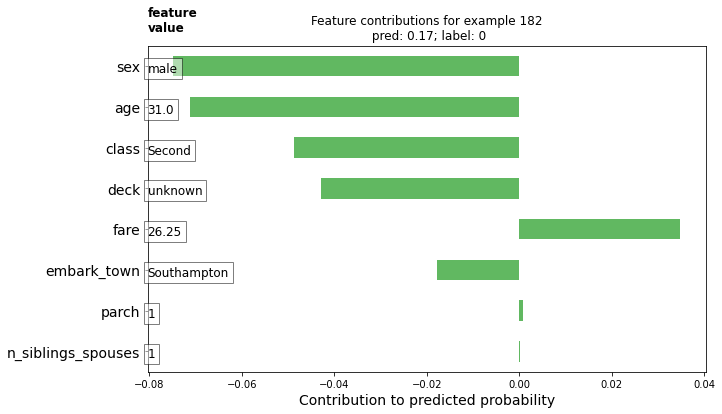The larger magnitude contributions have a larger impact on the model's prediction. Negative contributions indicate the feature value for this given example reduced the model's prediction, while positive values contribute an increase in the prediction.

You can also plot the example's DFCs compare with the entire distribution using a voilin plot.

# Boilerplate plotting code.
def dist_violin_plot(df_dfc, ID):
# Initialize plot.
fig, ax = plt.subplots(1, 1, figsize=(10, 6))

# Create example dataframe.
TOP_N = 8  # View top 8 features.
example = df_dfc.iloc[ID]
ix = example.abs().sort_values()[-TOP_N:].index
example = example[ix]
example_df = example.to_frame(name='dfc')

# Add contributions of entire distribution.
parts=ax.violinplot([df_dfc[w] for w in ix],
vert=False,
showextrema=False,
widths=0.7,
positions=np.arange(len(ix)))
face_color = sns_colors
alpha = 0.15
for pc in parts['bodies']:
pc.set_facecolor(face_color)
pc.set_alpha(alpha)

ax.scatter(example,
np.arange(example.shape),
color=sns.color_palette(),
s=100,
marker="s",
label='contributions for example')

# Legend
# Proxy plot, to show violinplot dist on legend.
ax.plot([0,0], [1,1], label='eval set contributions\ndistributions',
color=face_color, alpha=alpha, linewidth=10)
legend = ax.legend(loc='lower right', shadow=True, fontsize='x-large',
frameon=True)
legend.get_frame().set_facecolor('white')

# Format plot.
ax.set_yticks(np.arange(example.shape))
ax.set_yticklabels(example.index)
ax.grid(False, axis='y')
ax.set_xlabel('Contribution to predicted probability', size=14)


Plot this example.

dist_violin_plot(df_dfc, ID)
plt.title('Feature contributions for example {}\n pred: {:1.2f}; label: {}'.format(ID, probs[ID], labels[ID]))
plt.show()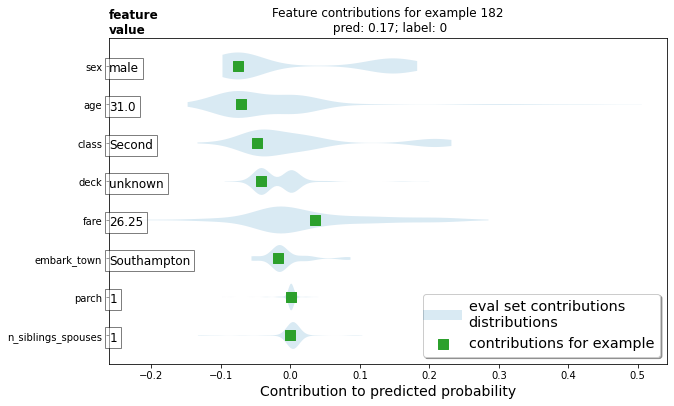Finally, third-party tools, such as LIME and shap, can also help understand individual predictions for a model.

## Global feature importances

Additionally, you might want to understand the model as a whole, rather than studying individual predictions. Below, you will compute and use:

• Gain-based feature importances using est.experimental_feature_importances
• Permutation importances
• Aggregate DFCs using est.experimental_predict_with_explanations

Gain-based feature importances measure the loss change when splitting on a particular feature, while permutation feature importances are computed by evaluating model performance on the evaluation set by shuffling each feature one-by-one and attributing the change in model performance to the shuffled feature.

In general, permutation feature importance are preferred to gain-based feature importance, though both methods can be unreliable in situations where potential predictor variables vary in their scale of measurement or their number of categories and when features are correlated (source). Check out this article for an in-depth overview and great discussion on different feature importance types.

### Gain-based feature importances

Gain-based feature importances are built into the TensorFlow Boosted Trees estimators using est.experimental_feature_importances.

importances = est.experimental_feature_importances(normalize=True)
df_imp = pd.Series(importances)

# Visualize importances.
N = 8
ax = (df_imp.iloc[0:N][::-1]
.plot(kind='barh',
color=sns_colors,
title='Gain feature importances',
figsize=(10, 6)))
ax.grid(False, axis='y')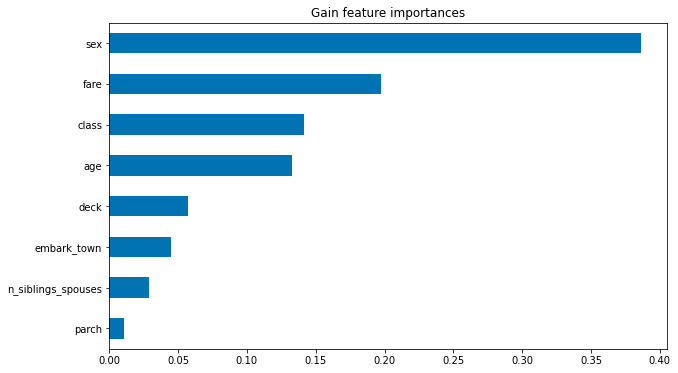### Average absolute DFCs

You can also average the absolute values of DFCs to understand impact at a global level.

# Plot.
dfc_mean = df_dfc.abs().mean()
N = 8
sorted_ix = dfc_mean.abs().sort_values()[-N:].index  # Average and sort by absolute.
ax = dfc_mean[sorted_ix].plot(kind='barh',
color=sns_colors,
title='Mean |directional feature contributions|',
figsize=(10, 6))
ax.grid(False, axis='y')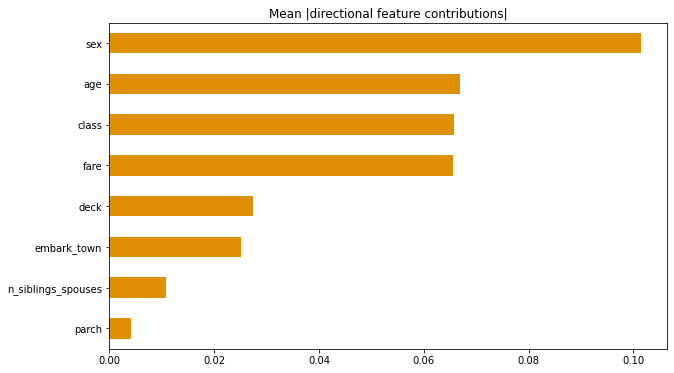You can also see how DFCs vary as a feature value varies.

FEATURE = 'fare'
feature = pd.Series(df_dfc[FEATURE].values, index=dfeval[FEATURE].values).sort_index()
ax = sns.regplot(feature.index.values, feature.values, lowess=True)
ax.set_ylabel('contribution')
ax.set_xlabel(FEATURE)
ax.set_xlim(0, 100)
plt.show()

/home/kbuilder/.local/lib/python3.7/site-packages/seaborn/_decorators.py:43: FutureWarning: Pass the following variables as keyword args: x, y. From version 0.12, the only valid positional argument will be data, and passing other arguments without an explicit keyword will result in an error or misinterpretation.
FutureWarning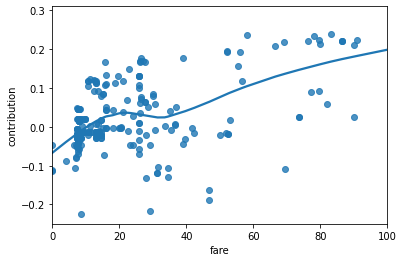### Permutation feature importance

def permutation_importances(est, X_eval, y_eval, metric, features):
"""Column by column, shuffle values and observe effect on eval set.

source: http://explained.ai/rf-importance/index.html
A similar approach can be done during training. See "Drop-column importance"
in the above article."""
baseline = metric(est, X_eval, y_eval)
imp = []
for col in features:
save = X_eval[col].copy()
X_eval[col] = np.random.permutation(X_eval[col])
m = metric(est, X_eval, y_eval)
X_eval[col] = save
imp.append(baseline - m)
return np.array(imp)

def accuracy_metric(est, X, y):
"""TensorFlow estimator accuracy."""
eval_input_fn = make_input_fn(X,
y=y,
shuffle=False,
n_epochs=1)
return est.evaluate(input_fn=eval_input_fn)['accuracy']
features = CATEGORICAL_COLUMNS + NUMERIC_COLUMNS
importances = permutation_importances(est, dfeval, y_eval, accuracy_metric,
features)
df_imp = pd.Series(importances, index=features)

sorted_ix = df_imp.abs().sort_values().index
ax = df_imp[sorted_ix][-5:].plot(kind='barh', color=sns_colors, figsize=(10, 6))
ax.grid(False, axis='y')
ax.set_title('Permutation feature importance')
plt.show()

INFO:tensorflow:Calling model_fn.
WARNING:tensorflow:Trapezoidal rule is known to produce incorrect PR-AUCs; please switch to "careful_interpolation" instead.
WARNING:tensorflow:Trapezoidal rule is known to produce incorrect PR-AUCs; please switch to "careful_interpolation" instead.
INFO:tensorflow:Done calling model_fn.
INFO:tensorflow:Starting evaluation at 2021-06-22T01:22:01
INFO:tensorflow:Graph was finalized.
INFO:tensorflow:Restoring parameters from /tmp/tmp5m737ngz/model.ckpt-153
INFO:tensorflow:Running local_init_op.
INFO:tensorflow:Done running local_init_op.
Exception ignored in: <function CapturableResource.__del__ at 0x7f50f8597cb0>
Traceback (most recent call last):
File "/tmpfs/src/tf_docs_env/lib/python3.7/site-packages/tensorflow/python/training/tracking/tracking.py", line 269, in __del__
with self._destruction_context():
AttributeError: 'TreeEnsemble' object has no attribute '_destruction_context'
INFO:tensorflow:Inference Time : 0.46432s
INFO:tensorflow:Finished evaluation at 2021-06-22-01:22:01
INFO:tensorflow:Saving dict for global step 153: accuracy = 0.81439394, accuracy_baseline = 0.625, auc = 0.86923784, auc_precision_recall = 0.85286695, average_loss = 0.41441453, global_step = 153, label/mean = 0.375, loss = 0.41441453, precision = 0.7604167, prediction/mean = 0.38847554, recall = 0.7373737
INFO:tensorflow:Saving 'checkpoint_path' summary for global step 153: /tmp/tmp5m737ngz/model.ckpt-153
INFO:tensorflow:Calling model_fn.
WARNING:tensorflow:Trapezoidal rule is known to produce incorrect PR-AUCs; please switch to "careful_interpolation" instead.
WARNING:tensorflow:Trapezoidal rule is known to produce incorrect PR-AUCs; please switch to "careful_interpolation" instead.
INFO:tensorflow:Done calling model_fn.
INFO:tensorflow:Starting evaluation at 2021-06-22T01:22:02
INFO:tensorflow:Graph was finalized.
INFO:tensorflow:Restoring parameters from /tmp/tmp5m737ngz/model.ckpt-153
INFO:tensorflow:Running local_init_op.
INFO:tensorflow:Done running local_init_op.
Exception ignored in: <function CapturableResource.__del__ at 0x7f50f8597cb0>
Traceback (most recent call last):
File "/tmpfs/src/tf_docs_env/lib/python3.7/site-packages/tensorflow/python/training/tracking/tracking.py", line 269, in __del__
with self._destruction_context():
AttributeError: 'TreeEnsemble' object has no attribute '_destruction_context'
INFO:tensorflow:Inference Time : 0.45788s
INFO:tensorflow:Finished evaluation at 2021-06-22-01:22:02
INFO:tensorflow:Saving dict for global step 153: accuracy = 0.625, accuracy_baseline = 0.625, auc = 0.66029996, auc_precision_recall = 0.54186726, average_loss = 0.7320349, global_step = 153, label/mean = 0.375, loss = 0.7320349, precision = 0.5, prediction/mean = 0.39807576, recall = 0.5252525
INFO:tensorflow:Saving 'checkpoint_path' summary for global step 153: /tmp/tmp5m737ngz/model.ckpt-153
INFO:tensorflow:Calling model_fn.
WARNING:tensorflow:Trapezoidal rule is known to produce incorrect PR-AUCs; please switch to "careful_interpolation" instead.
WARNING:tensorflow:Trapezoidal rule is known to produce incorrect PR-AUCs; please switch to "careful_interpolation" instead.
INFO:tensorflow:Done calling model_fn.
INFO:tensorflow:Starting evaluation at 2021-06-22T01:22:03
INFO:tensorflow:Graph was finalized.
INFO:tensorflow:Restoring parameters from /tmp/tmp5m737ngz/model.ckpt-153
INFO:tensorflow:Running local_init_op.
INFO:tensorflow:Done running local_init_op.
Exception ignored in: <function CapturableResource.__del__ at 0x7f50f8597cb0>
Traceback (most recent call last):
File "/tmpfs/src/tf_docs_env/lib/python3.7/site-packages/tensorflow/python/training/tracking/tracking.py", line 269, in __del__
with self._destruction_context():
AttributeError: 'TreeEnsemble' object has no attribute '_destruction_context'
INFO:tensorflow:Inference Time : 0.46375s
INFO:tensorflow:Finished evaluation at 2021-06-22-01:22:04
INFO:tensorflow:Saving dict for global step 153: accuracy = 0.8030303, accuracy_baseline = 0.625, auc = 0.85984075, auc_precision_recall = 0.83279574, average_loss = 0.4373517, global_step = 153, label/mean = 0.375, loss = 0.4373517, precision = 0.7326733, prediction/mean = 0.3994781, recall = 0.74747473
INFO:tensorflow:Saving 'checkpoint_path' summary for global step 153: /tmp/tmp5m737ngz/model.ckpt-153
INFO:tensorflow:Calling model_fn.
WARNING:tensorflow:Trapezoidal rule is known to produce incorrect PR-AUCs; please switch to "careful_interpolation" instead.
WARNING:tensorflow:Trapezoidal rule is known to produce incorrect PR-AUCs; please switch to "careful_interpolation" instead.
INFO:tensorflow:Done calling model_fn.
INFO:tensorflow:Starting evaluation at 2021-06-22T01:22:04
INFO:tensorflow:Graph was finalized.
INFO:tensorflow:Restoring parameters from /tmp/tmp5m737ngz/model.ckpt-153
INFO:tensorflow:Running local_init_op.
INFO:tensorflow:Done running local_init_op.
Exception ignored in: <function CapturableResource.__del__ at 0x7f50f8597cb0>
Traceback (most recent call last):
File "/tmpfs/src/tf_docs_env/lib/python3.7/site-packages/tensorflow/python/training/tracking/tracking.py", line 269, in __del__
with self._destruction_context():
AttributeError: 'TreeEnsemble' object has no attribute '_destruction_context'
INFO:tensorflow:Inference Time : 0.45918s
INFO:tensorflow:Finished evaluation at 2021-06-22-01:22:05
INFO:tensorflow:Saving dict for global step 153: accuracy = 0.81439394, accuracy_baseline = 0.625, auc = 0.86758494, auc_precision_recall = 0.8484707, average_loss = 0.41787332, global_step = 153, label/mean = 0.375, loss = 0.41787332, precision = 0.7604167, prediction/mean = 0.3886618, recall = 0.7373737
INFO:tensorflow:Saving 'checkpoint_path' summary for global step 153: /tmp/tmp5m737ngz/model.ckpt-153
INFO:tensorflow:Calling model_fn.
WARNING:tensorflow:Trapezoidal rule is known to produce incorrect PR-AUCs; please switch to "careful_interpolation" instead.
WARNING:tensorflow:Trapezoidal rule is known to produce incorrect PR-AUCs; please switch to "careful_interpolation" instead.
INFO:tensorflow:Done calling model_fn.
INFO:tensorflow:Starting evaluation at 2021-06-22T01:22:05
INFO:tensorflow:Graph was finalized.
INFO:tensorflow:Restoring parameters from /tmp/tmp5m737ngz/model.ckpt-153
INFO:tensorflow:Running local_init_op.
INFO:tensorflow:Done running local_init_op.
Exception ignored in: <function CapturableResource.__del__ at 0x7f50f8597cb0>
Traceback (most recent call last):
File "/tmpfs/src/tf_docs_env/lib/python3.7/site-packages/tensorflow/python/training/tracking/tracking.py", line 269, in __del__
with self._destruction_context():
AttributeError: 'TreeEnsemble' object has no attribute '_destruction_context'
INFO:tensorflow:Inference Time : 0.45474s
INFO:tensorflow:Finished evaluation at 2021-06-22-01:22:06
INFO:tensorflow:Saving dict for global step 153: accuracy = 0.75, accuracy_baseline = 0.625, auc = 0.7973064, auc_precision_recall = 0.7058313, average_loss = 0.5520768, global_step = 153, label/mean = 0.375, loss = 0.5520768, precision = 0.6813187, prediction/mean = 0.38672423, recall = 0.6262626
INFO:tensorflow:Saving 'checkpoint_path' summary for global step 153: /tmp/tmp5m737ngz/model.ckpt-153
INFO:tensorflow:Calling model_fn.
WARNING:tensorflow:Trapezoidal rule is known to produce incorrect PR-AUCs; please switch to "careful_interpolation" instead.
WARNING:tensorflow:Trapezoidal rule is known to produce incorrect PR-AUCs; please switch to "careful_interpolation" instead.
INFO:tensorflow:Done calling model_fn.
INFO:tensorflow:Starting evaluation at 2021-06-22T01:22:06
INFO:tensorflow:Graph was finalized.
INFO:tensorflow:Restoring parameters from /tmp/tmp5m737ngz/model.ckpt-153
INFO:tensorflow:Running local_init_op.
INFO:tensorflow:Done running local_init_op.
Exception ignored in: <function CapturableResource.__del__ at 0x7f50f8597cb0>
Traceback (most recent call last):
File "/tmpfs/src/tf_docs_env/lib/python3.7/site-packages/tensorflow/python/training/tracking/tracking.py", line 269, in __del__
with self._destruction_context():
AttributeError: 'TreeEnsemble' object has no attribute '_destruction_context'
INFO:tensorflow:Inference Time : 0.46550s
INFO:tensorflow:Finished evaluation at 2021-06-22-01:22:07
INFO:tensorflow:Saving dict for global step 153: accuracy = 0.79545456, accuracy_baseline = 0.625, auc = 0.8523722, auc_precision_recall = 0.83783334, average_loss = 0.43543077, global_step = 153, label/mean = 0.375, loss = 0.43543077, precision = 0.74725276, prediction/mean = 0.3862282, recall = 0.68686867
INFO:tensorflow:Saving 'checkpoint_path' summary for global step 153: /tmp/tmp5m737ngz/model.ckpt-153
INFO:tensorflow:Calling model_fn.
WARNING:tensorflow:Trapezoidal rule is known to produce incorrect PR-AUCs; please switch to "careful_interpolation" instead.
WARNING:tensorflow:Trapezoidal rule is known to produce incorrect PR-AUCs; please switch to "careful_interpolation" instead.
INFO:tensorflow:Done calling model_fn.
INFO:tensorflow:Starting evaluation at 2021-06-22T01:22:07
INFO:tensorflow:Graph was finalized.
INFO:tensorflow:Restoring parameters from /tmp/tmp5m737ngz/model.ckpt-153
INFO:tensorflow:Running local_init_op.
INFO:tensorflow:Done running local_init_op.
Exception ignored in: <function CapturableResource.__del__ at 0x7f50f8597cb0>
Traceback (most recent call last):
File "/tmpfs/src/tf_docs_env/lib/python3.7/site-packages/tensorflow/python/training/tracking/tracking.py", line 269, in __del__
with self._destruction_context():
AttributeError: 'TreeEnsemble' object has no attribute '_destruction_context'
INFO:tensorflow:Inference Time : 0.46516s
INFO:tensorflow:Finished evaluation at 2021-06-22-01:22:08
INFO:tensorflow:Saving dict for global step 153: accuracy = 0.8219697, accuracy_baseline = 0.625, auc = 0.87453324, auc_precision_recall = 0.85081327, average_loss = 0.41087124, global_step = 153, label/mean = 0.375, loss = 0.41087124, precision = 0.7888889, prediction/mean = 0.37933567, recall = 0.7171717
INFO:tensorflow:Saving 'checkpoint_path' summary for global step 153: /tmp/tmp5m737ngz/model.ckpt-153
INFO:tensorflow:Calling model_fn.
WARNING:tensorflow:Trapezoidal rule is known to produce incorrect PR-AUCs; please switch to "careful_interpolation" instead.
WARNING:tensorflow:Trapezoidal rule is known to produce incorrect PR-AUCs; please switch to "careful_interpolation" instead.
INFO:tensorflow:Done calling model_fn.
INFO:tensorflow:Starting evaluation at 2021-06-22T01:22:08
INFO:tensorflow:Graph was finalized.
INFO:tensorflow:Restoring parameters from /tmp/tmp5m737ngz/model.ckpt-153
INFO:tensorflow:Running local_init_op.
INFO:tensorflow:Done running local_init_op.
Exception ignored in: <function CapturableResource.__del__ at 0x7f50f8597cb0>
Traceback (most recent call last):
File "/tmpfs/src/tf_docs_env/lib/python3.7/site-packages/tensorflow/python/training/tracking/tracking.py", line 269, in __del__
with self._destruction_context():
AttributeError: 'TreeEnsemble' object has no attribute '_destruction_context'
INFO:tensorflow:Inference Time : 0.46522s
INFO:tensorflow:Finished evaluation at 2021-06-22-01:22:09
INFO:tensorflow:Saving dict for global step 153: accuracy = 0.81439394, accuracy_baseline = 0.625, auc = 0.86923784, auc_precision_recall = 0.85286695, average_loss = 0.41441453, global_step = 153, label/mean = 0.375, loss = 0.41441453, precision = 0.7604167, prediction/mean = 0.38847554, recall = 0.7373737
INFO:tensorflow:Saving 'checkpoint_path' summary for global step 153: /tmp/tmp5m737ngz/model.ckpt-153
INFO:tensorflow:Calling model_fn.
WARNING:tensorflow:Trapezoidal rule is known to produce incorrect PR-AUCs; please switch to "careful_interpolation" instead.
WARNING:tensorflow:Trapezoidal rule is known to produce incorrect PR-AUCs; please switch to "careful_interpolation" instead.
INFO:tensorflow:Done calling model_fn.
INFO:tensorflow:Starting evaluation at 2021-06-22T01:22:10
INFO:tensorflow:Graph was finalized.
INFO:tensorflow:Restoring parameters from /tmp/tmp5m737ngz/model.ckpt-153
INFO:tensorflow:Running local_init_op.
INFO:tensorflow:Done running local_init_op.
Exception ignored in: <function CapturableResource.__del__ at 0x7f50f8597cb0>
Traceback (most recent call last):
File "/tmpfs/src/tf_docs_env/lib/python3.7/site-packages/tensorflow/python/training/tracking/tracking.py", line 269, in __del__
with self._destruction_context():
AttributeError: 'TreeEnsemble' object has no attribute '_destruction_context'
INFO:tensorflow:Inference Time : 0.45312s
INFO:tensorflow:Finished evaluation at 2021-06-22-01:22:10
INFO:tensorflow:Saving dict for global step 153: accuracy = 0.7689394, accuracy_baseline = 0.625, auc = 0.7923477, auc_precision_recall = 0.77950954, average_loss = 0.49962917, global_step = 153, label/mean = 0.375, loss = 0.49962917, precision = 0.72619045, prediction/mean = 0.37482148, recall = 0.61616164
INFO:tensorflow:Saving 'checkpoint_path' summary for global step 153: /tmp/tmp5m737ngz/model.ckpt-153
INFO:tensorflow:Calling model_fn.
WARNING:tensorflow:Trapezoidal rule is known to produce incorrect PR-AUCs; please switch to "careful_interpolation" instead.
WARNING:tensorflow:Trapezoidal rule is known to produce incorrect PR-AUCs; please switch to "careful_interpolation" instead.
INFO:tensorflow:Done calling model_fn.
INFO:tensorflow:Starting evaluation at 2021-06-22T01:22:11
INFO:tensorflow:Graph was finalized.
INFO:tensorflow:Restoring parameters from /tmp/tmp5m737ngz/model.ckpt-153
INFO:tensorflow:Running local_init_op.
INFO:tensorflow:Done running local_init_op.
Exception ignored in: <function CapturableResource.__del__ at 0x7f50f8597cb0>
Traceback (most recent call last):
File "/tmpfs/src/tf_docs_env/lib/python3.7/site-packages/tensorflow/python/training/tracking/tracking.py", line 269, in __del__
with self._destruction_context():
AttributeError: 'TreeEnsemble' object has no attribute '_destruction_context'
INFO:tensorflow:Inference Time : 0.44399s
INFO:tensorflow:Finished evaluation at 2021-06-22-01:22:11
INFO:tensorflow:Saving dict for global step 153: accuracy = 0.7916667, accuracy_baseline = 0.625, auc = 0.8557392, auc_precision_recall = 0.8428282, average_loss = 0.43396166, global_step = 153, label/mean = 0.375, loss = 0.43396166, precision = 0.73913044, prediction/mean = 0.38084388, recall = 0.68686867
INFO:tensorflow:Saving 'checkpoint_path' summary for global step 153: /tmp/tmp5m737ngz/model.ckpt-153## Visualizing model fitting

Lets first simulate/create training data using the following formula:

$$z=x* e^{-x^2 - y^2}$$

Where (z) is the dependent variable you are trying to predict and (x) and (y) are the features.

from numpy.random import uniform, seed
from scipy.interpolate import griddata

# Create fake data
seed(0)
npts = 5000
x = uniform(-2, 2, npts)
y = uniform(-2, 2, npts)
z = x*np.exp(-x**2 - y**2)
xy = np.zeros((2,np.size(x)))
xy = x
xy = y
xy = xy.T

# Prep data for training.
df = pd.DataFrame({'x': x, 'y': y, 'z': z})

xi = np.linspace(-2.0, 2.0, 200),
yi = np.linspace(-2.1, 2.1, 210),
xi,yi = np.meshgrid(xi, yi)

df_predict = pd.DataFrame({
'x' : xi.flatten(),
'y' : yi.flatten(),
})
predict_shape = xi.shape

def plot_contour(x, y, z, **kwargs):
# Grid the data.
plt.figure(figsize=(10, 8))
# Contour the gridded data, plotting dots at the nonuniform data points.
CS = plt.contour(x, y, z, 15, linewidths=0.5, colors='k')
CS = plt.contourf(x, y, z, 15,
vmax=abs(zi).max(), vmin=-abs(zi).max(), cmap='RdBu_r')
plt.colorbar()  # Draw colorbar.
# Plot data points.
plt.xlim(-2, 2)
plt.ylim(-2, 2)


You can visualize the function. Redder colors correspond to larger function values.

zi = griddata(xy, z, (xi, yi), method='linear', fill_value='0')
plot_contour(xi, yi, zi)
plt.scatter(df.x, df.y, marker='.')
plt.title('Contour on training data')
plt.show()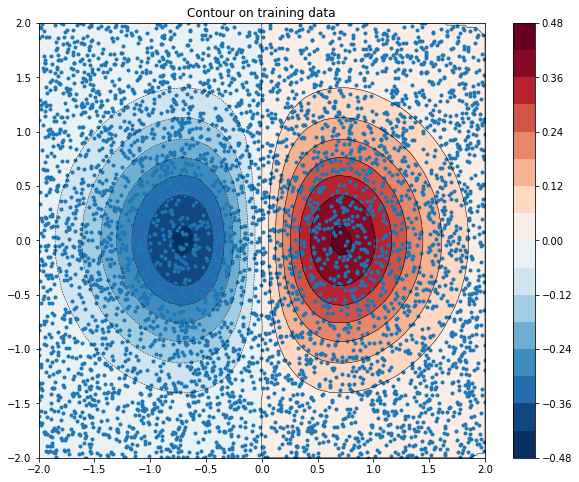fc = [tf.feature_column.numeric_column('x'),
tf.feature_column.numeric_column('y')]

def predict(est):
"""Predictions from a given estimator."""
predict_input_fn = lambda: tf.data.Dataset.from_tensors(dict(df_predict))
preds = np.array([p['predictions'] for p in est.predict(predict_input_fn)])
return preds.reshape(predict_shape)


First let's try to fit a linear model to the data.

train_input_fn = make_input_fn(df, df.z)
est = tf.estimator.LinearRegressor(fc)
est.train(train_input_fn, max_steps=500);

INFO:tensorflow:Using default config.
WARNING:tensorflow:Using temporary folder as model directory: /tmp/tmpmxyzf7fx
INFO:tensorflow:Using config: {'_model_dir': '/tmp/tmpmxyzf7fx', '_tf_random_seed': None, '_save_summary_steps': 100, '_save_checkpoints_steps': None, '_save_checkpoints_secs': 600, '_session_config': allow_soft_placement: true
graph_options {
rewrite_options {
meta_optimizer_iterations: ONE
}
}
, '_keep_checkpoint_max': 5, '_keep_checkpoint_every_n_hours': 10000, '_log_step_count_steps': 100, '_train_distribute': None, '_device_fn': None, '_protocol': None, '_eval_distribute': None, '_experimental_distribute': None, '_experimental_max_worker_delay_secs': None, '_session_creation_timeout_secs': 7200, '_checkpoint_save_graph_def': True, '_service': None, '_cluster_spec': ClusterSpec({}), '_task_type': 'worker', '_task_id': 0, '_global_id_in_cluster': 0, '_master': '', '_evaluation_master': '', '_is_chief': True, '_num_ps_replicas': 0, '_num_worker_replicas': 1}
INFO:tensorflow:Calling model_fn.
WARNING:tensorflow:From /tmpfs/src/tf_docs_env/lib/python3.7/site-packages/tensorflow/python/keras/optimizer_v2/ftrl.py:149: calling Constant.__init__ (from tensorflow.python.ops.init_ops) with dtype is deprecated and will be removed in a future version.
Instructions for updating:
Call initializer instance with the dtype argument instead of passing it to the constructor
/tmpfs/src/tf_docs_env/lib/python3.7/site-packages/tensorflow/python/keras/engine/base_layer_v1.py:1700: UserWarning: layer.add_variable is deprecated and will be removed in a future version. Please use layer.add_weight method instead.
warnings.warn('layer.add_variable is deprecated and '
INFO:tensorflow:Done calling model_fn.
INFO:tensorflow:Create CheckpointSaverHook.
INFO:tensorflow:Graph was finalized.
INFO:tensorflow:Running local_init_op.
INFO:tensorflow:Done running local_init_op.
INFO:tensorflow:Calling checkpoint listeners before saving checkpoint 0...
INFO:tensorflow:Saving checkpoints for 0 into /tmp/tmpmxyzf7fx/model.ckpt.
INFO:tensorflow:Calling checkpoint listeners after saving checkpoint 0...
INFO:tensorflow:loss = 0.025694462, step = 0
INFO:tensorflow:global_step/sec: 337.357
INFO:tensorflow:loss = 0.018777132, step = 100 (0.297 sec)
INFO:tensorflow:global_step/sec: 385.9
INFO:tensorflow:loss = 0.01891744, step = 200 (0.259 sec)
INFO:tensorflow:global_step/sec: 377.986
INFO:tensorflow:loss = 0.017629504, step = 300 (0.264 sec)
INFO:tensorflow:global_step/sec: 383.271
INFO:tensorflow:loss = 0.018930735, step = 400 (0.261 sec)
INFO:tensorflow:Calling checkpoint listeners before saving checkpoint 500...
INFO:tensorflow:Saving checkpoints for 500 into /tmp/tmpmxyzf7fx/model.ckpt.
INFO:tensorflow:Calling checkpoint listeners after saving checkpoint 500...
INFO:tensorflow:Loss for final step: 0.018376777.
<tensorflow_estimator.python.estimator.canned.linear.LinearRegressorV2 at 0x7f50c02cd9d0>

plot_contour(xi, yi, predict(est))

INFO:tensorflow:Calling model_fn.
INFO:tensorflow:Done calling model_fn.
INFO:tensorflow:Graph was finalized.
INFO:tensorflow:Restoring parameters from /tmp/tmpmxyzf7fx/model.ckpt-500
INFO:tensorflow:Running local_init_op.
INFO:tensorflow:Done running local_init_op.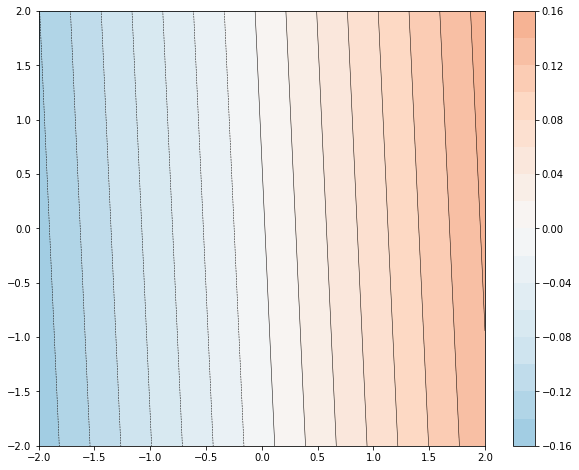It's not a very good fit. Next let's try to fit a GBDT model to it and try to understand how the model fits the function.

n_trees = 37

est = tf.estimator.BoostedTreesRegressor(fc, n_batches_per_layer=1, n_trees=n_trees)
est.train(train_input_fn, max_steps=500)
clear_output()
plot_contour(xi, yi, predict(est))
plt.text(-1.8, 2.1, '# trees: {}'.format(n_trees), color='w', backgroundcolor='black', size=20)
plt.show()

INFO:tensorflow:Calling model_fn.
INFO:tensorflow:Done calling model_fn.
INFO:tensorflow:Graph was finalized.
INFO:tensorflow:Restoring parameters from /tmp/tmp27_g75ww/model.ckpt-222
INFO:tensorflow:Running local_init_op.
INFO:tensorflow:Done running local_init_op.
Exception ignored in: <function CapturableResource.__del__ at 0x7f50f8597cb0>
Traceback (most recent call last):
File "/tmpfs/src/tf_docs_env/lib/python3.7/site-packages/tensorflow/python/training/tracking/tracking.py", line 269, in __del__
with self._destruction_context():
AttributeError: 'TreeEnsemble' object has no attribute '_destruction_context'As you increase the number of trees, the model's predictions better approximates the underlying function.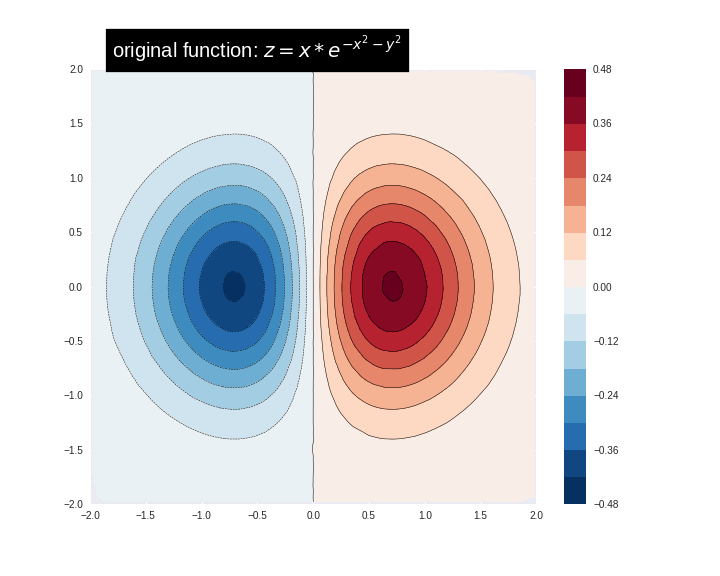## Conclusion

In this tutorial you learned how to interpret Boosted Trees models using directional feature contributions and feature importance techniques. These techniques provide insight into how the features impact a model's predictions. Finally, you also gained intution for how a Boosted Tree model fits a complex function by viewing the decision surface for several models.

[]
[]